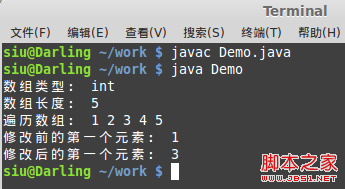# 基于java中反射的总结分析

2020-03-17 23:12

x_x 在此又痛恨一边那些厚部头们，把我的脑细胞搞死一片

Class类

package org.siu;

class Test {

}

public class Demo {
public static void main(String[] args) {
Test t = new Test();
System.out.println(t.getClass());
System.out.println(t.getClass().getName());
}
}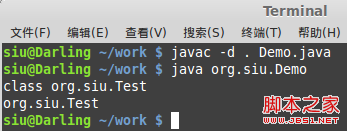（不过我想说的是，此处的泛型设计很刺眼！尼玛，整个java的语法设计同样刺眼，超恶心！！！）

forName( )

class Test {

}

public class Demo {
public static void main(String[] args) {
//方式一：
Test t = new Test();
Class<? extends Test> c1 = t.getClass();
System.out.println(c1);

//方式二：
//为了避免特殊性，这里不用Test类，而用java库中的String类
Class<String> c2 = String.class;
System.out.println(c2);

//方式三：
//forName()方法会抛出异常
Class<?> c3 = null;
try {
c3 = Class.forName("Test");
} catch (ClassNotFoundException e) {
e.printStackTrace();
}
System.out.println(c3);
}
}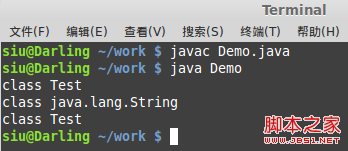Class类的应用

Class类中有一个方法叫做newInstance( ),它可以用来创建一个Class类对象的新实例

public class Demo {
public static void main(String[] args) {
//实例化Class对象，forName()方法会抛异常
Class<?> c = null;
try {
//这里需要完整的包名和类名
c = Class.forName("java.lang.String");
} catch (ClassNotFoundException e) {
e.printStackTrace();
}

//生成一个字符串的引用
String s = null;
try {
//将构造好的对象向下转型为String类
//newInstance()方法会抛异常
s = (String) c.newInstance();
} catch (InstantiationException e) {
e.printStackTrace();
} catch (IllegalAccessException e) {
e.printStackTrace();
}
System.out.println("字符串长度： " + s.length());
}
}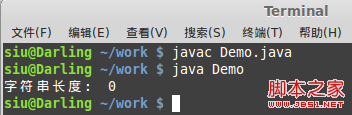import java.lang.reflect.Constructor;

public class Demo {
//下面的几个方法抛出来的异常太多，为了代码的紧凑性，这里就直接抛给虚拟机了
public static void main(String[] args) throws Exception {
Class<?> c = null;
try {
c = Class.forName("java.lang.String");
} catch (ClassNotFoundException e) {
e.printStackTrace();
}
char[] ch = {'h','e','l','l','o'};
String s = null;
//获得Class类对象的有参构造方法，括号里面参数的写法是：类型.class
Constructor<?> con = c.getConstructor(char[].class);
//用此构造方法构造一个新的字符串对象，参数为一个char数组
s = (String) con.newInstance(ch);
System.out.println("构造的字符串：" + s);
}
}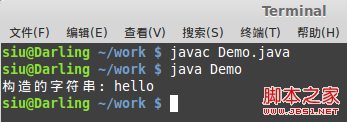import java.lang.reflect.Constructor;
import java.util.Arrays;

public class Demo {
//下面的几个方法抛出来的异常太多，为了代码的紧凑性，这里就直接抛给虚拟机了
public static void main(String[] args) throws Exception {
Class<?> c = null;
try {
c = Class.forName("java.lang.Boolean");
} catch (ClassNotFoundException e) {
e.printStackTrace();
}
//这里的getConstructors()方法返回的是一个Constructor数组
Constructor<?>[] cons = c.getConstructors();
//打印的方式你可以自己写，为了方便我用Arrays.toString()，凑合着看
System.out.println(Arrays.toString(cons));
}
}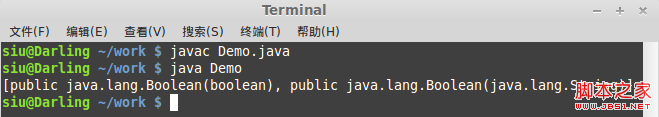import java.util.Arrays;

public class Demo {
public static void main(String[] args) throws Exception {
Class<?> c = null;
try {
c = Class.forName("java.lang.Boolean");
} catch (ClassNotFoundException e) {
e.printStackTrace();
}
Class<?>[] in = c.getInterfaces();
System.out.println(Arrays.toString(in));
}
}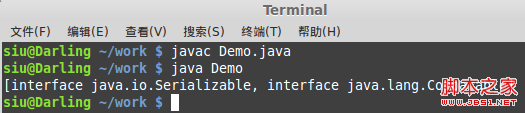public class Demo {
public static void main(String[] args) throws Exception {
Class<?> c = null;
try {
c = Class.forName("java.lang.Boolean");
} catch (ClassNotFoundException e) {
e.printStackTrace();
}
//注意了，这里不会是数组，why？
Class<?> su = c.getSuperclass();
System.out.println(su);
}
}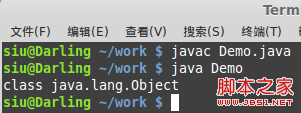import java.lang.reflect.Method;

public class Demo {
public static void main(String[] args) throws Exception {
Class<?> c = null;
try {
c = Class.forName("java.lang.Boolean");
} catch (ClassNotFoundException e) {
e.printStackTrace();
}
Method[] m = c.getMethods();
//好吧，这次我就大发慈悲的写个打印列表出来
for (int i = 0; i < m.length; i++) {
System.out.println(m[i]);
}
}
}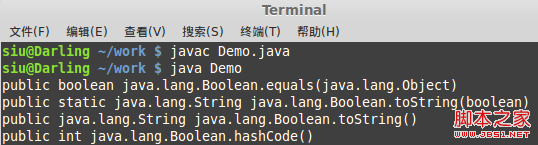import java.lang.reflect.Field;

class Person {
private String name;
private int age;
}

public class Demo {
public static void main(String[] args) throws Exception {
Class<?> c = null;
try {
c = Class.forName("Person");
} catch (ClassNotFoundException e) {
e.printStackTrace();
}
Field[] f = c.getDeclaredFields();
for (int i = 0; i < f.length; i++) {
System.out.println(f[i]);
}
}
}

getDeclaredFielsd()方法可以获取全部属性，getFields()只能获取公共属性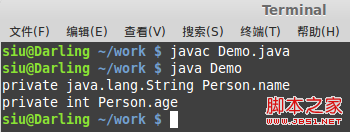import java.lang.reflect.Field;

class Person {
public String name;
private int age;

public Person(String name, int age) {
this.name = name;
this.age = age;
}
}

public class Demo {
public static void main(String[] args) throws Exception {
Person p = new Person("zhangsan",12);

Class<?> c = p.getClass();

//获取公共属性的值
Field f1 = c.getField("name");
//get(p)表明要获取是哪个对象的值
String str = (String) f1.get(p);
System.out.println("姓名： " + str);

//获取私有属性的值
Field f2 = c.getDeclaredField("age");
//age是私有属性，所以要设置安全检查为true
f2.setAccessible(true);
int age = (int) f2.get(p);
System.out.println("年龄： " + age);
}
}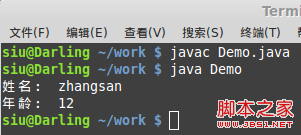import java.lang.reflect.Field;

class Person {
private String name;

public Person(String name) {
this.name = name;
}

public String toString() {
return "姓名： " + this.name;
}
}

public class Demo {
public static void main(String[] args) throws Exception {
Person p = new Person("王二狗");
System.out.println(p);
Class<?> c = p.getClass();

//定义要修改的属性
Field f = c.getDeclaredField("name");
f.setAccessible(true);
//修改属性，传入要设置的对象和值
f.set(p, "张二蛋");
System.out.println(p);
}
}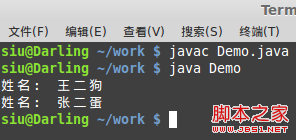import java.lang.reflect.Method;

class Person {
public void print(int i) {
System.out.println("我在写数字： " + i);
}

public static void say(String str) {
System.out.println("我在说： " + str);
}
}

public class Demo {
public static void main(String[] args) throws Exception {
Person p = new Person();
Class<?> c = p.getClass();

//getMethod()方法需要传入方法名，和参数类型
Method m1 = c.getMethod("print", int.class);
//invoke()表示调用的意思，需要传入对象和参数
m1.invoke(p, 10);

Method m2 = c.getMethod("say", String.class);
//这里的null表示不由对象调用，也就是静态方法
m2.invoke(null, "你妹");
}
}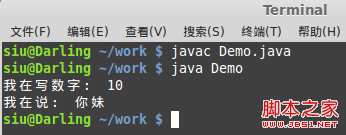import java.lang.reflect.Array;

public class Demo {
public static void main(String[] args) throws Exception {
int[] arr = {1,2,3,4,5};
Class<?> c = arr.getClass().getComponentType();

System.out.println("数组类型： " + c.getName());
int len = Array.getLength(arr);
System.out.println("数组长度： " + len);
System.out.print("遍历数组： ");
for (int i = 0; i < len; i++) {
System.out.print(Array.get(arr, i) + " ");
}
System.out.println();
//修改数组
System.out.println("修改前的第一个元素： " + Array.get(arr, 0));
Array.set(arr, 0, 3);
System.out.println("修改后的第一个元素： " + Array.get(arr, 0));
}
}